# Redefining statistical significance: the statistical arguments

## Part two of a three part series

[Part one of this series is here]

At the heart of the RSS paper are a number of statistical arguments. There are three, and I will address each of them in this (rather long) post. They are 1) two-sided p values around .05 are evidentially weak, 2) using a lower α would decrease the so-called “false discovery rate,” and 3) empirical evidence from large scale replications shows that studies with p<.005 are more likely to replicate.

As I said in the previous post, I don’t have a problem with α=.005 per se. I believe that the arguments for it are weak, and worse, they muddle debates over methods. If we are to build a successful science on the rubble of the past, clarity is the most important thing right now.

Here’s a summary of the points:

1. Bayes factors can overstate the evidence relative to p values, depending on the way one sets up the test. Even under the best possible (realistic) scenario for the RSS team — assuming their arguments, but using the best analysis — the resulting p value calibration turns out to be .02–.03, not .005.
2. The “false discovery rate” is a flawed concept: it is an ill-defined rate based on a misunderstanding of statistical power.
3. The “empirical” demonstration that studies with p values <.005 are more likely to replicate is not an empirical finding at all; it is a necessary property of a positive correlation. It would occur with any pair of α levels, and hence could be used to argue that α should be made arbitrarily small. It cannot be used as an argument for α=.005.

To begin, I’ll flip the RSS paper’s argument on its head and show that one can easily argue that Bayes factors overstate evidence when compared to p values.

## Do Bayes factors overstate the statistical evidence?

Suppose we would like to assess the evidence for the sign of an effect using a significance test: we’re interested in the whether the true mean of a normal population is greater than or less than 100. The population has known standard deviation of 15, and we sample N=250. We observe =98.55, for z=-1.53, and we reason:

“This result is inconsistent with a non-negative effect, and consistent with a negative effect. How inconsistent with a non-negative effect is it? If the effect size were truly non-negative, we would seldom, but not very rarely, see negative effect sizes greater than this one (p=.063, or 1 time in 15). If I were to say z=-1.53 is strong evidence for a negative effect, I certainly would call z≤-1.53 strong evidence for a negative effect. Under these circumstances, I could potentially err 6% of the time (e.g., when the effect size is 0 or just above).

“If I were to accept z≤-1.53 as strong evidence for a negative effect, I should also accept z≥1.53 as strong evidence for a positive effect. Again, I would err about 6% of the time (when the effect size is 0 or just below). To account for the flexibility of naming the sign after seeing the data, I report a total error rate of twice .063, or p=.126.

“If I were to use criterion of |z|≥1.53 to claim I have strong evidence for the sign of the effect, I could potentially be misled 13% of the time (when the effect has neither sign). This is often enough that I should not claim that |z|≥1.53 is strong evidence for one sign or the other.”

(See Mayo and Cox, 2006, for an outline of significance testing logic, including the underlying philosophical principle of evidence.)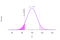Figure 1. Computing a p value in the example. The distribution shown is the sampling distribution for X̅ assuming that μ=100. The observed X̅ is at least 1.53 standard errors below where it would be expected if μ≥100.

Figure 1 shows how the p value was computed relative to the sampling distribution assuming μ=100, which is the most extreme element of the null hypothesis when testing the alternative that μ>100 and that μ<100.

It is not my purpose here to advocate or refute this logic. I will say that almost every statistician and scientist I know has a mixture of Bayesian and frequentist intuitions, and even most Bayesians would find the basic principle that “strong evidence should not be often misleading” (as I would paraphrase Mayo and Cox’s principle) intuitive. Although one has the choice to accept or reject the principle, that choice will be based on other intuitions about evidence one might have.

Under the current standards of evidence in psychology, a p value of .133 is not impressive. Let’s now consider the Bayes factor for the negative effect sizes versus positive effect sizes under assumptions similar to those of the RSS team. We know that (apologies for Medium’s terrible math notation support):

~ Normal(μ, σ/√250 = .949).

We need a prior for μ. For the sake of the example, suppose that we choose:

μ ~ Normal(100, 5).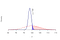Figure 2. Prior and posterior for the example. The prior is the red dashed line, with the prior probability that μ>100 shaded in red (.5). The posterior is shown in blue, with the posterior probability that μ>100 shaded in blue (.066).

The prior is depicted as the red dashed distribution in Figure 2.

Our posterior will be

μ|X̅ ~ Normal(98.6, .932).

The posterior probability that μ<100 is 93.4%. The Bayes factor for the comparison that μ<100 versus μ≥100 is

.934 / (1-.934) = 14.

(The Bayes factor is the same as the posterior odds because the prior is symmetric around 100, and hence the prior odds are 1.)

This Bayes factor of 14 is within the range that Jeffreys — and prominent members of the RSS team following him —would claim represents “strong” evidence.¹ Bayesians following these evidential guidelines would claim that we have strong evidence that the sign of the effect is negative (versus positive; Bayes factors are always relative). But the p value is .13, which most researchers would regard as insufficient evidence. In fact, Jeffreys’ guidelines on evidence call Bayes factors above 10 “strong”. In this example, a Bayes factor of 10 corresponds to a z value of -1.36 and a p value of .174. I don’t know anyone who would claim that p≈.174 is strong evidence for anything. Shall we echo the RSS team and say that we suspect many scientists would guess that BF≈10 implies stronger support for the sign of an effect than a p value of .174? Shall we call for a change to Bayesian practice to bring them in line with p values?

How could our conclusion above differ so much from the RSS team? What’s going on?

As the RSS team notes, “There is no unique mapping between the P value and the Bayes factor, since the Bayes factor depends on H₁.” The Bayes factor depends not only on H₁, but also on the choice of H₀. This is where all the action is.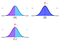Figure 3. A: Dividing the continuous parameter space into two parts; e.g., positive and negative effect sizes. One part can be tested against the other. B: A point null against a two-sided alternative. Tests “nothing” against “something”. C: The parameter space is divided into two continuous hypotheses and a point null is added. Three comparisons are now possible: positive versus “nothing”, negative versus “nothing”, and positive versus negative.

Bayesian analysis is flexible with respect to the types of hypotheses one can test. Figure 3 shows a number of ways of setting up a hypothesis test for a parameter of interest. The example above used the setup in Figure 3(A), and came to the conclusion that a Bayes factor of 10 is weaker evidence than a p value of about .05. The RSS team uses the setup in Figure 3 (B), mistakenly thinking that a Bayesian two-sided test is analogous to a classical two-sided test. We now explore this confusion.

The purpose of significance testing. It is taken for granted by the RSS team that the purpose of significance testing is to test a point null hypothesis against a two-sided alternative. The authors translate this into a two-sided Bayesian test with a point null. But if one doesn’t assent to their interpretation of the purpose of the p value, then the link between the p value and their particular assessments of the Bayesian evidence falls apart, and the argument with it.

Consider, for instance, if instead of viewing the “two-sided” significance test as such, we understood it as two one-sided tests with a correction (doubling the p value). This is arguably a better way of understanding significance testing; it certainly aligns with many of its proponents’ views, as well as giving the p value a Bayesian interpretation under some conditions.

In comparison, the Bayesian two-sided test is artificial; under what circumstances would a scientist care about an effect but not its sign? The Bayesian one-sided solution discussed above is arguably less conservative than the p value, because it does not correct for post hoc selection of the sign to be tested. Bayesians can, and do, argue that from a Bayesian perspective one should not correct, but then a frequentist is free to argue — consistently with their conception of evidence related to error rates — that any Bayesian ignoring cherry-picking is overstating the evidence.

If the purpose of a significance test is testing one sign against the other, then as we have shown, the Bayes factor — not the p value — can appear to overstate the evidence. Wagenmakers has argued that p values are intended as point-null tests against two-sided alternatives, and hence we should evaluate them as such, but it seems like building a reform around the expectations of badly-trained scientists might be a bad idea.

The use of a point null. A key aspect of the argument made by the RSS team is that a p value is weak evidence against the null, which to them is a point null (e.g., μ=100, exactly) in their Bayesian model. I will not argue that this is never appropriate. But it is enough to say 1) this has no effect on the frequentist p value, so claiming that a point null test is the purpose of a significance test is suspect, and 2) many Bayesians do not believe this is appropriate in all, or even most, situations.

In a significance test, the null and alternative will be complementary; when one is testing whether there is evidence to claim that μ>100, the appropriate “foil” (or “null”) for this is that μ≤100. The fact that the p value is computed under the worst-case scenario — μ=100 — might make one believe that a significance test is a test of a point null, but it needn’t be thought of that way. But importantly, the p value doesn’t change whichever way you look at it.

Under the Bayesian model, however, it matters a great deal whether the null is μ≤100 or μ=100, and how much prior probability we assign to μ=100. For a Bayesian, this is as it should be. It is precisely this flexibility that Bayesians will point to as a benefit of Bayesian analysis, pointing out that “if you ask a different question, then you should get a different answer.”

So how, then, did a group of Bayesians become so normative about the use of a point null? EJ Wagenmakers explains:

“[E]mpirical claims often concern the presence of a phenomenon. In such situations, any reasonable skeptic will remain unconvinced when the data fail to discredit the point-null.”

But this fails to account for the fact that, under this view,

1. the hypothetical skeptic must be a Bayesian, because the argument doesn’t work otherwise,
2. this particular (Bayesian) skeptic must always be assuaged (but only for users of p values; see below), and
3. one is licensed to ignore frequentist skeptics, because the conception of evidence used for the standard does not address frequentist concerns (like multiple tests).

It sounds good to address skeptical claims, but this isn’t really about skepticism per se: if it were multiple avenues of legitimate skepticism would be addressed. This is about the normativity of a particular conception of statistical evidence under a set of restrictive model assumptions.

To further emphasise this point Stephen Senn has pointed out that there isn’t really about a disagreement between p values and Bayes factors; it is really a disagreement between Bayesian evidence when a point null is included and Bayesian evidence when it is not. It is not the case that p values overstate evidence, because they were never meant to state Bayesian evidence. It is the case that Bayesian evidence computed with respect to a particular set of hypotheses will differ from Bayesian evidence computed with respect to another set of hypotheses. The wisdom of incorporating a null hypothesis will depend on the situation, and Bayesian analysts can disagree. As Rouder, Morey, and Wagenmakers argued elsewhere (just last year!):

“It is necessary to instantiate theory as a set of competing models, and this instantiation is a creative, innovative, value-added activity. A diversity of models even for the same theory should be embraced as part of an intellectual richness rather than be the subject of some arbitrary homogenization under the euphemism of convention.” [emphasis not in original]

Given that this disagreement is between Bayesians, the RSS’s teams statement that “restrict [the] recommendation to studies that conduct null hypothesis significance tests” is especially strange. The RSS team is suggesting that Bayesians can get away with lax Bayesian evidential standards (by not using a point null), but users of p values, which were never intended to be Bayesian evidence, cannot. This is a curious recommendation indeed.

The main point, though, is that under some reasonable priors the p value will appear to “overstate” the Bayesian evidence; under others, the opposite. There is no general way to use Bayesian statistics to calibrate p values. We now turn to the choice of the RSS team to use two-sided Bayesian tests rather than one-sided, and the implication this choice has for their evidential threshold.

The unreasonableness of two-sided alternatives. The RSS authors state that “[The evidence] can be evaluated for particular test statistics under certain classes of plausible alternatives.” We’ve already examined the role of the null hypothesis in the argument, and how different choices completely change the assessment of evidence (as is typical of Bayesian statistics). We now examine the alternatives used in Figure 3.

As already mentioned, the Bayesian alternative hypotheses used by the RSS team are all two-sided. This is an strange choice, as any data analyst would care about the sign of the effect. Imagine being told that a researcher was studying an intervention and they could tell you it has an effect, but they couldn’t tell you whether the intervention helped or hurt. As mentioned before, a classical two-sided test is just two one-sided tests with a (frequentist) correction for opportunistic selection of the sign. So a frequentist has no problem naming the sign of the effect. But a Bayesian comparing the null to a two-sided alternative would.

There’s an easy Bayesian answer: a Bayesian can chop the alternative into halves at the null, and do two one-sided tests (see Figure 3, C). Conveniently, in common situations (including the ones considered by the RSS team) the relationship between the two-sided Bayes factor and the best of two one-sided Bayes factors is approximately a factor of two. There is no cost to this for a Bayesian, because a Bayesian doesn’t have to correct for doing both tests, unlike a frequentist. This changes the relationship between the Bayesian evidence of interest to an analyst and the p value, because we can obtain strong evidence for hypotheses of interest for much larger p values.

I’ll explain the steps.

1. Compute the two-sided Bayes factor. Call it B. This is the relative evidence between the two-sided alternative and the point null.
2. Consider the alternative only. The prior odds for the effects of either sign individually compared to the whole two-sided alternative are 1/2 (because the prior is symmetric).
3. Again, consider the alternative only and consider the sign that is consistent with the data. As is well-known, symmetric, sufficiently diffuse priors, the p value is related to the posterior probability that the effect has that sign. The posterior probability is approximately 1-p/2 (see Morey and Wagenmakers).
4. To obtain the Bayes factor for the sign consistent with the data against the full, two-sided alternative, we divide the posterior odds (from 3 above) by the prior odds (from 2 above), which gives 2-p. We’ll call this Bayes factor A.
5. Since B is a comparison between the two-sided alternative and the point null, and A is a comparison between the sign consistent with the observed effect and the two-sided alternative, we can use the transitivity of the Bayes factor to compute the Bayes factor comparing the sign consistent with the data against the point null by multiplying B by A. The resulting one-sided Bayes factor is just (2-p)B.
6. We could repeat the logic above for the sign inconsistent with the data, if we liked.
7. We note that for significant (low) p values, 2-p is about 2.

The basic idea outlined above is that we can compute a one-sided Bayes factor from a two-sided one by “boosting” the evidence in favor of the sign that was consistent with the data. The correction factor will be related to the posterior probability of that sign. Under the models described in the RSS team’s paper, for significant p values the correction factor will be about 2. When the RSS team says we have two-sided Bayes factor of about 5 — which they would not call “strong” evidence — we actually know we have useful, one-sided Bayes factor of about 10, which they would call “strong”.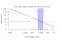Figure 4. An adaptation of the RSS team’s Figure 1, plotting the Bayes factor in favor of the data-consistent hypothesis against the point null. The four calibrations yield “strong” evidence for two-sided p values between about .02 and .03. You can also view an interactive version of this figure.

The implications for their argument are immediate. We can adapt the RSS team’s Figure 1, which shows the relationship between the Bayes factor and the two sided p value for four calibrations. Figure 4 here shows the one-sided Bayes factors — in favor of the hypothesis consistent with the data — for each of these four calibrations. The shaded region shows the range of p values that yield “strong” evidence. In the worst case scenario, the local-H₁ bound, a “strong” one-sided Bayes factor of 10 corresponds to a p value of about .02; in the best case, about .03. (This interactive version of this Figure 4 allows you to specify your own calibration. Try it out.)

So even under the assumptions of the RSS team, in making an inference about the sign of the effect against a point null the most extreme recalibration for significance is p<.02.² I suspect the suggestion to redefine significance to p<.02-.03 would have captured much less attention than redefining it to .005.

Suppose that we accept the argument of the RSS team, with the added proviso that a Bayesian should be using one-sided priors because they can at no cost. We arrive at the conclusion that two-sided p values between .03 and .05 are not “strong” Bayesian evidence (according to Jeffreys), and perhaps also two-sided p values between .02 and .03 (according to the RSS calibrations, p=.02 corresponds to a one-sided Bayes factor of between 9 and 15). If we adopt the proposal to redefine these “weak” p values between .02 and .05 to suggestive rather than significant, what proportion of results would be affected? As shown in Figure 5, this depends on the true effect size and the sample size. To even get the probability over 15% requires a rather unlucky choice of sample size. For many combinations, the probability is in the single-digit percents.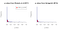Figure 6. Histograms of observed p values collected by Wetzels et al (2011) and Hartgerink (2016). The red bars show the ones that would be affected by a redefinition based on insufficient Bayesian evidence (.02

We can also look at large collections of statistics from empirical papers. Wetzels et al (2011) collected 855 t tests from psychology journals and Hartgerink (2016) collected 686,200 test statistics from psychology journals. Figure 6 shows the empirical distributions of the corresponding p values. Only about 14% of the p values are in the problematic range of .02<p≤.05 in both sets. This is undoubtedly inflated by publication bias and p hacking, neither of which the RSS team’s proposal addresses. The percentage of p values in the problematic range observed by these published researchers may be in the single digits.

Taking all of this together, does it make sense to attribute problems of replicability to the weak Bayesian evidence of two-sided p values between .005 and .05? Probably not. As we have seen, p values between .005 and .05 need not offer weak evidence, even under the assumptions of the RSS authors. Further, the proportion of p values in the range that might be problematic under their assumptions appears to be small.

Any change based on a reasonable implementation of the logic of the RSS team (that is, disregarding .005 and going with, say, .02), even if fully implemented, is likely to have little impact.

## “Evidence” from false discovery rates

The second argument marshalled by the RSS team is that lowering α to .005 will lower so-called “false discovery rates”. Deborah Mayo and I have a relevant paper (preprint) where we critique the false discovery rate. Basically: there is no false discovery rate. There cannot be, because the scientific process cannot be analogized to drawing random “hypotheses” from an urn, some of which are true and some false. It is based on a complete misunderstanding of the concept of power. The RSS team have, unfortunately, promulgated these misunderstandings.

To fully appreciate the irony of a Bayesian paper using this argument, consider the way that Bayesian statistics is sold: it is a way to make an inference conditional on the data you obtained! You don’t need to consider any “long run” rate of significance of hypothetical replications. That’s great, right? But what’s the “False Discovery Rate”? It is a hypothetical rate, but computed against an ill-defined reference class (studies done in a “field”), and has nothing to do with evaluating the evidence from a study. It is worse than the rates (Type I errors, etc) that Bayesians have traditionally complained about, because the rate doesn’t even have a definable reference class.

The “false discovery rate” does not exist. When was the last time you pulled a random hypothesis from an urn and tested it? What would a random hypothesis even mean? Are hypotheses truly “null” or “non-null”? Is there a “power” associated with a “field”?

The answers are “never,” “nothing,” “no,” and “no.” The false discovery rate is a bizarre hybrid probability, computed using pseudo-Bayesian logic but masquerading as a frequentist rate. You can safely ignore any argument based on this flawed concept (or its complement, the PPV).

## “Empirical” evidence from large-scale replications

One of the more potentially interesting lines of evidence the RSS team claims for the proposal to reduce α to .005 is original studies that had p<.005 were more likely to be replicated. I’m most familiar with the Reproducibility Project: Psychology (RP:P), which they cite as support; so I’ll focus on that.

The RP:P collected a number of variables that allow us to evaluate their predictive value for the replications. For instance, we might want to see whether the original p value predicts the replication p value; or perhaps the assessed “surprisingness” of the original result.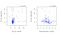Figure 7. Left: Correlation between the p value of the original paper and the p value of the replication. Right: Correlation between the rated surprisingness of the original study and the replication. p values have been transformed to Z statistics for visual clarity. Kendall’s nonparametric τ correlation is given at the top.

Figure 7(left panel) shows the correlation between the original study’s p value and the replication study’s p value. The relationship is positive (τ=.213), but not terribly impressive. The RSS team point out that for original studies that report p<.005, about half “replicated” by the RP:P’s p value criterion (interestingly, p<.05). For original studies that reported p>.005, only about a quarter of the replications yielded p<.05. Leaving aside the interesting irony that p<.05 is enough to show a replication, we note that although this is suggestive, it is also correlational. For now, though, we take it at face value.

Figure 7 (right) shows the correlation between the rated “surprisingness” of the original result and the replication p value. The relationship is negative (τ=-.241), and stronger than the relationship between the original and replication p values. If we set a criterion of suprisingness<3.06 (the mean suprisingness score), we get roughly the same dichotomous breakdown: for original studies that were “unsurprising” (suprisingness<3.06) about half “replicated.” For “surprising” original studies, only about a quarter of the replications yielded p<.05.

Focus just on the “surprisingness” result. What should we do with this information? Should we propose a formal “surpringness” label to go into articles alongside the results? The answer seems obvious (at least to me). We should do all the things that I described in my previous post. What else are we supposed to do, as scientists? The problem here is not “surprisingness” — sometimes we get surprising results, and that is ok: it’s actually what we hope for, because surprising results represent theoretical progress if they are robust. The problem is that many results — including surprising results — are not followed up on. Phenomena need to be understood, which requires repeated experimentation.

Likewise, a statistical significance criterion at .05 is not the problem (aside from publication bias, which the RSS team’s proposal does not address); the problem is that many results are not followed up on. Scientists take a difference in means as solid evidence for a phenomenon, and in turn take this as solid evidence for a theory. Both steps are hasty. The problem is at the level of philosophy of science, not verbal labels for p values.

Let us step back for a moment and examine the correlational nature of this supposed “empirical” evidence that the reduction would help replicability. Of course, a correlation between two quantities does not mean that if you change one quantity, the other will change with it, so we cannot really take the correlation as evidence. But there is something more deeply wrong with taking this correlation as empirical evidence. What the RSS team describes is not actually an empirical phenomenon; this correlation arises from a few assumptions to which everyone would agree. The claimed “benefit” to replicability will exist for any pair of α levels that one would care to choose, because this is how correlations work.

Consider two exam scores — say, a midterm and a final — that are correlated. We might note that people that got an A on the midterm were more likely to get above a B on the final than those who scored B or above on the midterm. Of course they are. That’s what a positive correlation means.

If we assume two things:

1. Across studies in large replication projects, true effect sizes between an original study and a replication correlate positively. (This is just the condition that they are, in fact, replications.)
2. p values are negatively correlated with true effect sizes: larger effect sizes, on average, lead to lower p values. (This is just the condition that experimenters are not completely incompetent or conspiring in some way to erase the correlation by examining small effect with gigantic sample sizes and large effects with very small sample sizes).

These two conditions will ensure that the p values for the original and replication are correlated. No one (I think) would dispute these conditions, and they are enough to ensure that original studies that yielded p<.005 (or any other p<.05) have a greater probability of seeing replications that yield p<.05. Of course they do. That’s what a positive correlation means.

If the instructor of our hypothetical class decided to change the criterion for an A on the midterm, do we have reason to believe this will improve student performance on the final? No.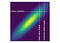Figure 8. With a positive correlation, conditioning on a more extreme criterion on one variable will ensure that a greater proportion of observations are extreme in the other variable.

From any starting criterion on p, if we decrease the criterion on p we will increase the probability of a replication having a low p value. This is shown visually in Figure 8. Again, that’s just how positive correlations work. This argument could be used to lower α to any arbitrarily low level; it can’t be used to support α=.005. But, you say, that’s ridiculous; surely the RSS team wouldn’t advocate that. They would consider the tradeoffs involved in lowering α…

And now you see the critic’s point of view.

## Conclusion

Each of the RSS team’s arguments for the adoption of a criterion of α=.005 fails under scrutiny.

• Bayes factors do not generally “overstate” the evidence offered by p values. Depending on the model, the opposite may appear to occur, and the evidential principle underlying p values is different anyway. To get to the conclusion of the RSS team, one must adopt a Bayesian view of evidence and specific prior assumptions, ignoring the richness of Bayesian inference.
• The RSS team’s use of two-sided alternatives exaggerated the problem, even under their assumptions. Bayesians have no need for two-sided alternatives, because they can use one-sided alternatives at no cost. This boosts the evidence to the data-consistent side by a factor of about 2. Using this fact, the calibration of the limits to “strong” evidence (10) is about p=.02 to .03 (the RSS authors give us nothing but upper bounds on the evidence to calibrate to). Even under the more defensible one-sided version of their argument, “weak” evidential p values account for a small minority results, so it seems difficult to believe that simply renaming them suggestive will have an effect.
• So-called “false discovery rates” don’t exist. Any such calculation is irrelevant to scientific practice.
• The “empirical” evidence from large scale replications is a simple truism about positive correlations. The truism cannot be used to argue for lowering α to .005; if it could, it could also be used to reduce α to any tiny value larger than 0. This would be absurd.

In the next post — the third in this series — I will explore the various responses to the proposal.

## Footnotes

¹ It isn’t clear what specific criteria the RSS team are using, but RSS author EJ Wagenmakers favors Jeffreys’ “10-as-strong-evidence” categorization and have used it in his own papers. See Table 1 from Andraszewicz et al (2015), and more recently Aczel et al, in preprint which states “a Bayes factor reanalysis revealed that fewer than 5% of the nonsignificant findings provided strong evidence (i.e., BF01 > 10)…”

² The RSS team could object at this point that the Bayes factor calibrations they suggest are based on upper bounds, and so calibrating based on a Bayes factor of 10 means that the corresponding p value of, say .02 will not be sufficient evidence under some actual prior. If we grant the RSS team the point null — which a Bayesian should not, because it should not be normative — then taking the priors historically suggested by the members of the RSS team, there is only one of the upper bounds that counts: the likelihood ratio, which is the most liberal of the upper bounds (and yields a criterion of over .03). Johnson and Rossell (2010) and for instance, advocate “non-local” priors that are not subject to the Local-H1 bound. Johnson (2013) suggests one-sided point priors, which again are not subject to the local-H1 bound. There is no reason why we should feel restricted by the conditions of the local-H1 bound, even assuming the point prior. This is even apart from the fact that all of the numbers suggested by the RSS team are arbitrary. It is my goal to show that a similar but more defensible line of argumentation can lead to different results.
Further, there is an inherent difficulty of arguing against a calibration based on an upper bound. It seems that you need to exceed the Bayes factor in the calibration, but by how much? It isn’t clear, because the actual Bayes factor will depend on the model and be informed by the context. The RSS team’s lowest Bayes factor is 14. Why? Was it simply that .005 is a nice number, and 14 just happened to be above 10? It isn’t clear. (You can see by using the interactive version of Figure 4 that for a Bayes factor of 14, the calibrations run from .012 to .022). Arguing about these numbers is like boxing shadows. If the RSS team were to make a principled argument, it might be “use Bayes factors informed by context.” But they didn’t; they instead tried to use Bayes factors to calibrate p values, which is impossible in general.

Written by